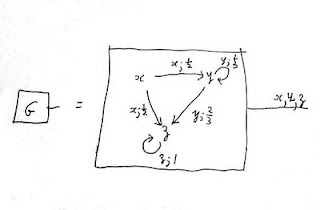Wednesday, April 13, 2011

Como Category Seminar: What does "observable" mean? Hidden Markov models

In various applications, for example in language recognition, there is a variation of the notion of Markov chain called the Hidden Markov model. The word "hidden" refers to the fact that the states are regarded as hidden or unobservable. Roughly the idea is this: the states are syntactical classes, and there is a given probability of passing from one class to another. Further, in a certain class there is a probability associated with each element of the class, the probability that this element is output, or visible.

The question of what is visible or not is just declared by the formal definition, but I would like to suggest that visible has a wider meaning. A state or a transition of an open system is observable or visible if it is the projection of a state or transition of the system on its boundary. What does boundary mean? The boundary is the part of the open system which may be glued to the boundary of another open system. In other words composition of open systems determines what observable means. What is observable is what can be joined to an observer. The extreme case is that closed systems have no visible features.

Let me give an equivalent but alternative definition of hidden markov model in which these ideas are explicit.  Consider the following open markov chain G in which the states are visible (as transitions on the boundary):Now compose this system with one V with only one state, and a transition for each element of each syntactic class (the same element may occur in different syntactic classes with different probabilities), for example:

The composed system is:

in which the states of the system G are hidden. Of course inferences may be made about them: one may ask, for example, given an observed string what is the probability that a certain path in the system G was used to generate it - a probabilistic parsing.
This system is pictured in the hidden markov model as:

In practice there are many fewer syntactic rules than words in the vocabulary, and it the separation of syntax from vocabulary which is presented as the advantage of the hidden markov model, a separation which is particularly clear in the compositional description.

Labels: